Home   »   Education News   »   Class 12 Maths Term 2 Answer...

# Class 12 Maths Term 2 Answer Key & Question Paper Solution

## CBSE Class 12th Term 2 Maths Answer Key

The Class 12 Term 2 Mathematics exam will be conducted between 10:30 am and 12:30 pm. Students can get the CBSE Class 12th Term 2 Mathematics Answer Key after 12:30 pm on this page. The miscellaneous information for the CBSE Class 12th Term 2 Mathematics Answer Key is provided in the table below.

 CBSE Class 12th Term 2 Mathematics Answer Key Exam Conducting Body Central Board of Secondary Education Exam & Subject Name CBSE Class 12 Mathematics Category Answer Key Exam Date 7th June 2022, Tuesday Unofficial Answer Key 7th June 2022, Tuesday Official Answer Key To be notified Official Website https://www.cbse.nic.in/

## Maths Class 12 Term 2 Answer Key and Exam Pattern

The exam pattern given here is based on the CBSE Class 12 Term 2 Mathematics sample paper released by the board on its official website. Class 12 Term 2 Mathematics holds 40 marks. The students will get 2 hours to complete the CBSE Class 12 Term 2 Mathematics question paper. The CBSE Class 12 Term 2 Mathematics question paper consists of three sections, namely Section A, Section B, and Section C. Check the other details given below:

Section A: This section consists of 6 short answer-type questions. Each question carries 2 marks.

Section B: This section consists of 4 short answer-type questions. Each question carries 3 marks.

Section C: This section consists of 4 long answer-type questions. Each question carries 4 marks.

Note: One case-based problem will be asked. The case-based question has 2 sub-parts of 2 marks each.

## Maths Class 12 Term 2 Question Paper for Answer Key

Maths Question Paper- 65/4/1

Maths Question Papers Set- 65/4/3

## Maths Class 12 Term 2 Answer Key: Paper Code 65/4/1

Section A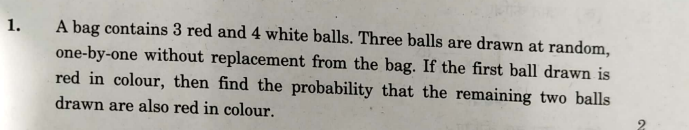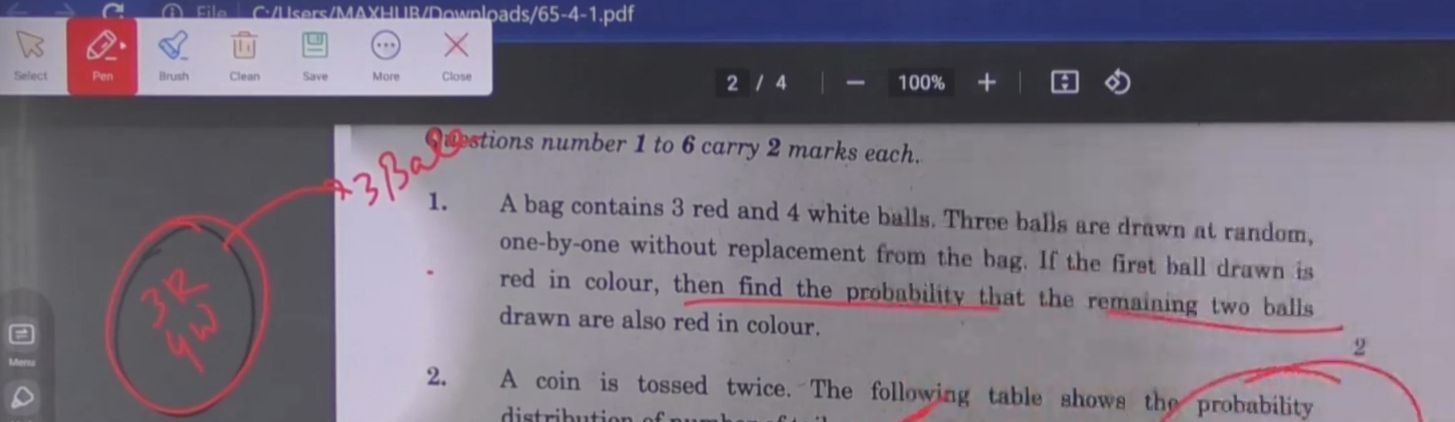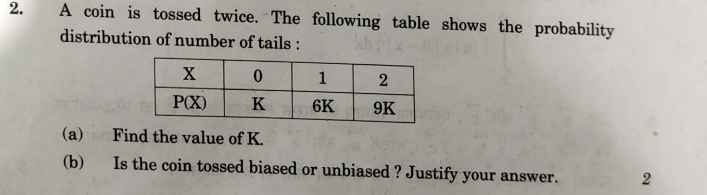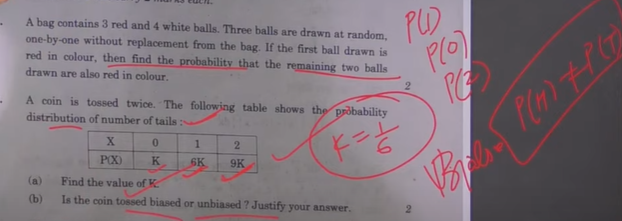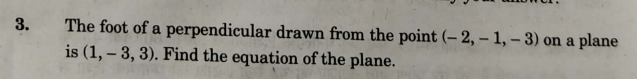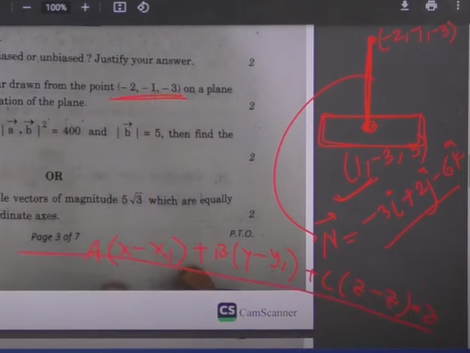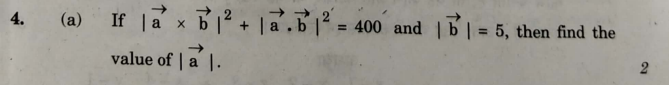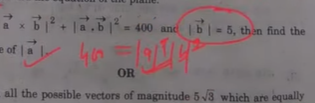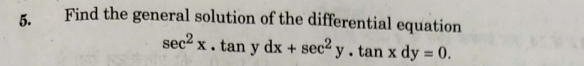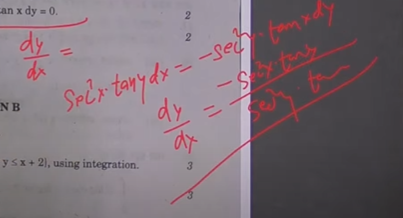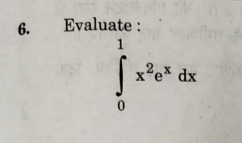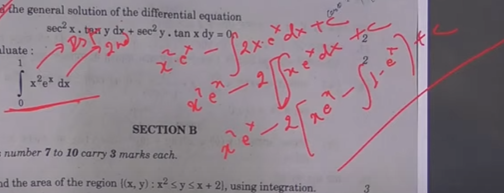Section B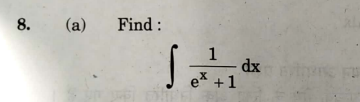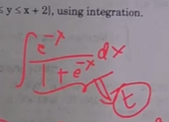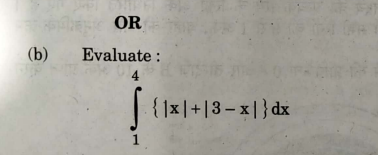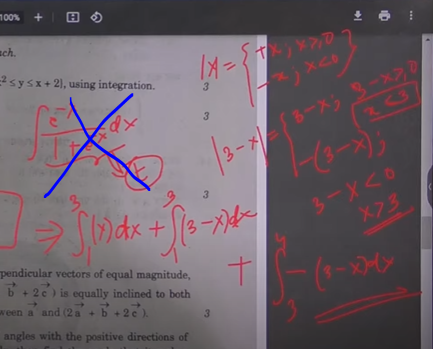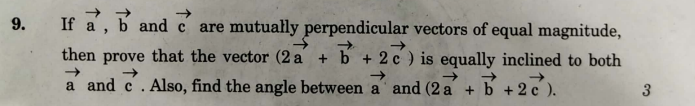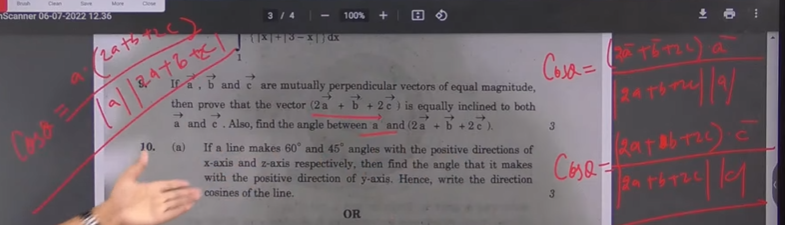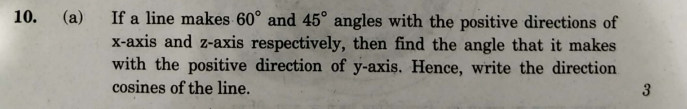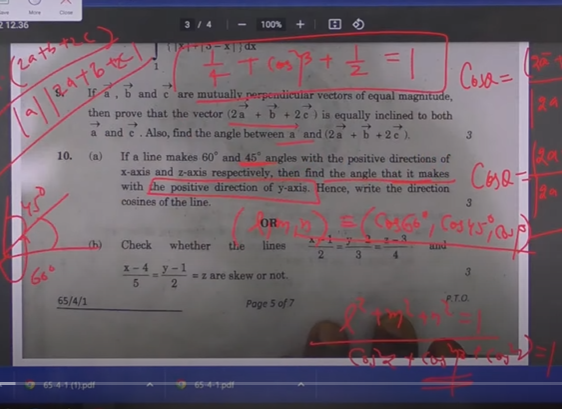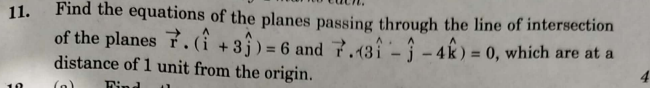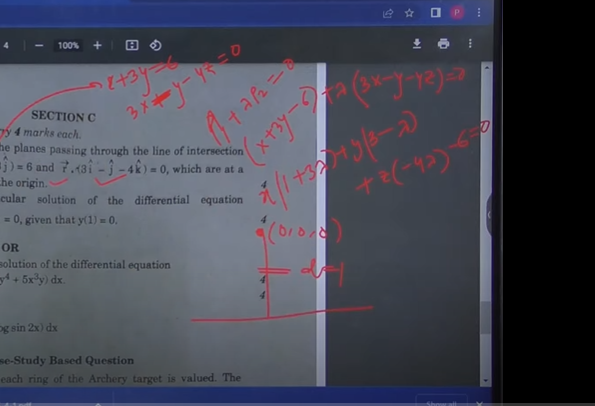## CBSE Class 12 Maths Term 2 Answer Key 2022: FAQs

Q. Where can I get the Class 12 Term 2 Mathematics Answer Key 2022?

Q. What is the subject code for Mathematics Class 12?

The subject code for Class 12 Mathematics is 041.

Q. What is the exam pattern for Class 12 Term 2 Mathematics?

The Class 12 Term 2 Mathematics question paper consists of 3 sections. Section A consists of short answer type questions of 2 marks each, Section B consists of short answer type questions of 3 marks each while Section C consists of long answer type questions of 4 marks each.

Sharing is caring!

Thank You, Your details have been submitted we will get back to you.